Next: Collisions in 2-dimensions Up: Conservation of momentum Previous: Impulses

## Collisions in 1-dimension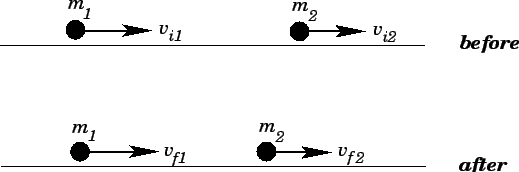Consider two objects of mass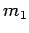and, respectively, which are free to move in 1-dimension. Suppose that these two objects collide. Suppose, further, that both objects are subject to zero net force when they are not in contact with one another. This situation is illustrated in Fig. 54.

Both before and after the collision, the two objects move with constant velocity. Let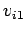and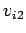be the velocities of the first and second objects, respectively, before the collision. Likewise, let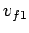and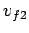be the velocities of the first and second objects, respectively, after the collision. During the collision itself, the first object exerts a large transitory force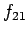on the second, whereas the second object exerts an equal and opposite force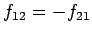on the first. In fact, we can model the collision as equal and opposite impulses given to the two objects at the instant in time when they come together.

We are clearly considering a system in which there is zero net external force (the forces associated with the collision are internal in nature). Hence, the total momentum of the system is a conserved quantity. Equating the total momenta before and after the collision, we obtain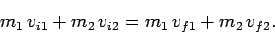(217)

This equation is valid for any 1-dimensional collision, irrespective its nature. Note that, assuming we know the masses of the colliding objects, the above equation only fully describes the collision if we are given the initial velocities of both objects, and the final velocity of at least one of the objects. (Alternatively, we could be given both final velocities and only one of the initial velocities.)

There are many different types of collision. An elastic collision is one in which the total kinetic energy of the two colliding objects is the same before and after the collision. Thus, for an elastic collision we can write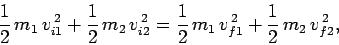(218)

in addition to Eq. (217). Hence, in this case, the collision is fully specified once we are given the two initial velocities of the colliding objects. (Alternatively, we could be given the two final velocities.)

The majority of collisions occurring in real life are not elastic in nature. Some fraction of the initial kinetic energy of the colliding objects is usually converted into some other form of energy--generally heat energy, or energy associated with the mechanical deformation of the objects--during the collision. Such collisions are termed inelastic. For instance, a large fraction of the initial kinetic energy of a typical automobile accident is converted into mechanical energy of deformation of the two vehicles. Inelastic collisions also occur during squash/racquetball/handball games: in each case, the ball becomes warm to the touch after a long game, because some fraction of the ball's kinetic energy of collision with the walls of the court has been converted into heat energy. Equation (217) remains valid for inelastic collisions--however, Eq. (218) is invalid. Thus, generally speaking, an inelastic collision is only fully characterized when we are given the initial velocities of both objects, and the final velocity of at least one of the objects. There is, however, a special case of an inelastic collision--called a totally inelastic collision--which is fully characterized once we are given the initial velocities of the colliding objects. In a totally inelastic collision, the two objects stick together after the collision, so that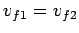.

Let us, now, consider elastic collisions in more detail. Suppose that we transform to a frame of reference which co-moves with the centre of mass of the system. The motion of a multi-component system often looks particularly simple when viewed in such a frame. Since the system is subject to zero net external force, the velocity of the centre of mass is invariant, and is given by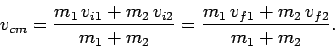(219)

An object which possesses a velocityin our original frame of reference--henceforth, termed the laboratory frame--possesses a velocity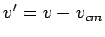in the centre of mass frame. It is easily demonstrated that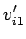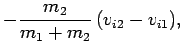(220)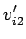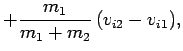(221)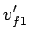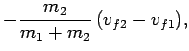(222)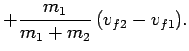(223)

The above equations yield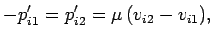(224)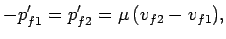(225)

where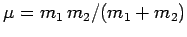is the so-called reduced mass, and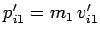is the initial momentum of the first object in the centre of mass frame, etc. In other words, when viewed in the centre of mass frame, the two objects approach one another with equal and opposite momenta before the collision, and diverge from one another with equal and opposite momenta after the collision. Thus, the centre of mass momentum conservation equation,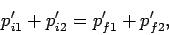(226)

is trivially satisfied, because both the left- and right-hand sides are zero. Incidentally, this result is valid for both elastic and inelastic collisions.

The centre of mass kinetic energy conservation equation takes the form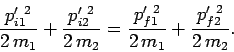(227)

Note, incidentally, that if energy and momentum are conserved in the laboratory frame then they must also be conserved in the centre of mass frame. A comparison of Eqs. (224), (225), and (227) yields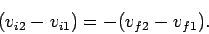(228)

In other words, the relative velocities of the colliding objects are equal and opposite before and after the collision. This is true in all frames of reference, since relative velocities are frame invariant. Note, however, that this result only applies to fully elastic collisions.

Equations (217) and (228) can be combined to give the following pair of equations which fully specify the final velocities (in the laboratory frame) of two objects which collide elastically, given their initial velocities: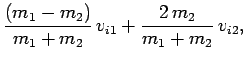(229)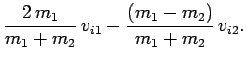(230)

Let us, now, consider some special cases. Suppose that two equal mass objects collide elastically. If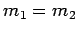then Eqs. (229) and (230) yield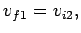(231)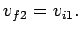(232)

In other words, the two objects simply exchange velocities when they collide. For instance, if the second object is stationary and the first object strikes it head-on with velocitythen the first object is brought to a halt whereas the second object moves off with velocity. It is possible to reproduce this effect in pool by striking the cue ball with great force in such a manner that it slides, rather that rolls, over the table--in this case, when the cue ball strikes another ball head-on it comes to a complete halt, and the other ball is propelled forward very rapidly. Incidentally, it is necessary to prevent the cue ball from rolling, because rolling motion is not taken into account in our analysis, and actually changes the answer.

Suppose that the second object is much more massive than the first (i.e.,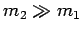) and is initially at rest (i.e.,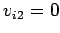). In this case, Eqs. (229) and (230) yield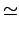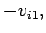(233)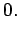(234)

In other words, the velocity of the light object is effectively reversed during the collision, whereas the massive object remains approximately at rest. Indeed, this is the sort of behaviour we expect when an object collides elastically with an immovable obstacle: e.g., when an elastic ball bounces off a brick wall.

Suppose, finally, that the second object is much lighter than the first (i.e.,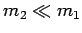) and is initially at rest (i.e.,). In this case, Eqs. (229) and (230) yield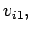(235)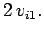(236)

In other words, the motion of the massive object is essentially unaffected by the collision, whereas the light object ends up going twice as fast as the massive one.

Let us, now, consider totally inelastic collisions in more detail. In a totally inelastic collision the two objects stick together after colliding, so they end up moving with the same final velocity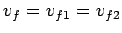. In this case, Eq. (217) reduces to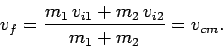(237)

In other words, the common final velocity of the two objects is equal to the centre of mass velocity of the system. This is hardly a surprising result. We have already seen that in the centre of mass frame the two objects must diverge with equal and opposite momenta after the collision. However, in a totally inelastic collision these two momenta must also be equal (since the two objects stick together). The only way in which this is possible is if the two objects remain stationary in the centre of mass frame after the collision. Hence, the two objects move with the centre of mass velocity in the laboratory frame.

Suppose that the second object is initially at rest (i.e.,). In this special case, the common final velocity of the two objects is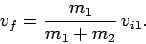(238)

Note that the first object is slowed down by the collision. The fractional loss in kinetic energy of the system due to the collision is given by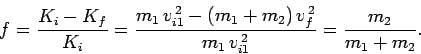(239)

The loss in kinetic energy is small if the (initially) stationary object is much lighter than the moving object (i.e., if), and almost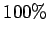if the moving object is much lighter than the stationary one (i.e., if). Of course, the lost kinetic energy of the system is converted into some other form of energy: e.g., heat energy.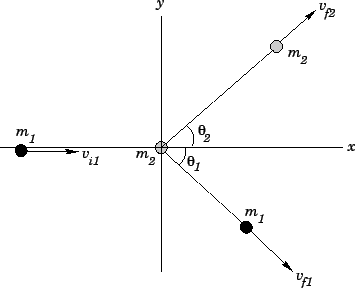Next: Collisions in 2-dimensions Up: Conservation of momentum Previous: Impulses
Richard Fitzpatrick 2006-02-02